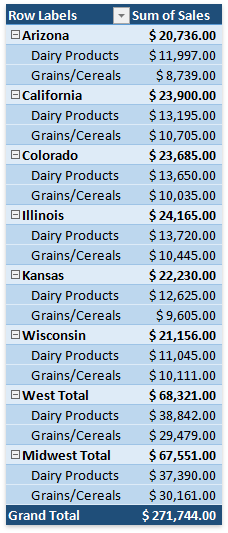20.1
19.2
19.1
18.2
18.1
17.2

# How to: Create a Calculated Item

If the predefined aggregation functions or Show Values As calculation options do not meet your requirements, you can create your own formulas to calculate values in a PivotTable report by inserting calculated fields and calculated items. A calculated item is a custom item in a PivotTable field whose value is produced based on values of other items in the same field.

All calculated items added to a PivotTable field are stored in the PivotCalculatedItemCollection collection, which can be accessed using the PivotField.CalculatedItems property. Use the collection's methods to create, modify or remove calculated items.

## Calculated Item Limitations

Before inserting a calculated item, take into account the following resrictions.

## Create a Calculated Item

To create a calculated item, use the PivotCalculatedItemCollection.Add method. The first parameter of this method allows you specify a formula for the calculated item.

A formula string should conform to the common syntax rules and contain only supported elements.

• In the formula, you can use constants and refer to other items in the same field where the calculated item resides. To create an item reference, use one of the approaches listed in the table below. All examples in the table refer to the pivot table shown later in this section.

Refere By

Description

Example

Item Name

Refer to an item by its name in the PivotTable field (PivotItem.Caption).

When creating a reference, you can enclose the item's name in apostrophes or omit them.

The following example demonstrates how to sum up the first three items in the "State" field:

...or...

Item Position

Refer to an item by its position in the PivotTable as currently sorted and displayed.

Hidden items are ignored.

The following example demonstrates how to sum up the first three items in the "State" field:

=State+State+State

Item Relative Position

Refer to an item by its position relative to the calculated item containing the formula.

Positive numbers refer to items below or to the right of the calculated item.

Negative numbers refer to items above or to the left of the calculated item.

If the specified position is before the first item or after the last item in the field, the #REF! error is displayed.

The following example demonstrates how to sum up the first three items in the "State" field relative to the "West Total" calculated item:

=State[-6]+State[-5]+State[-4]

• You cannot create formulas that use a cell reference, defined name, circular references and arrays.
• You cannot use worksheet functions that require cell references or defined names as arguments.
• The formula cannot refer to the PivotTable's subtotals, totals and Grand Total value.

The following code demonstrates how to create two calculated items to calculate total sales for each region.

##### NOTE

A complete sample project is available at https://github.com/DevExpress-Examples/wpf-spreadsheet-pivot-table-api-t314425.

``````Dim worksheet As Worksheet = workbook.Worksheets("Report10")
workbook.Worksheets.ActiveWorksheet = worksheet

' Access the pivot table by its name in the collection.
Dim pivotTable As PivotTable = worksheet.PivotTables("PivotTable1")

' Access the pivot field by its name in the collection.
Dim field As PivotField = pivotTable.Fields("State")

' Add calculated items to the "State" field.
``````

The resulting PivotTable report is shown in the image below.## Modify a Calculated Item

To change a formula for a calculated item, get access to the required item by its index in the PivotCalculatedItemCollection collection and then assign a new formula to the item's PivotItem.Formula property. To rename a calculated item, use the PivotItem.Caption property.

##### NOTE

A complete sample project is available at https://github.com/DevExpress-Examples/wpf-spreadsheet-pivot-table-api-t314425.

``````Dim worksheet As Worksheet = workbook.Worksheets("Report7")
workbook.Worksheets.ActiveWorksheet = worksheet

' Access the pivot table by its name in the collection.
Dim pivotTable As PivotTable = worksheet.PivotTables("PivotTable1")

' Access the pivot field by its name in the collection.
Dim field As PivotField = pivotTable.Fields("Customer")

' Add a calculated item to the "Customer" field.
Dim item As PivotItem = field.CalculatedItems.Add("='Big Foods'*110%", "Big Foods Sales Plan")

'Change the formula for the calculated item.
item.Formula = "='Big Foods'*115%"
``````

## Remove a Calculated Item

To remove a calculated item from the field, use the PivotCalculatedItemCollection.Remove or PivotCalculatedItemCollection.RemoveAt method. To remove all calculated items from the collection at once, use the PivotCalculatedItemCollection.Clear method.

##### NOTE

A complete sample project is available at https://github.com/DevExpress-Examples/wpf-spreadsheet-pivot-table-api-t314425.

``````Dim worksheet As Worksheet = workbook.Worksheets("Report7")
workbook.Worksheets.ActiveWorksheet = worksheet

' Access the pivot table by its name in the collection.
Dim pivotTable As PivotTable = worksheet.PivotTables("PivotTable1")

' Access the pivot field by its name in the collection.
Dim field As PivotField = pivotTable.Fields("Customer")

' Add a calculated item to the "Customer" field.
field.CalculatedItems.Add("='Big Foods'*110%", "Big Foods Sales Plan")

'Remove the calculated item by its index from the collection.
field.CalculatedItems.RemoveAt(0)
``````